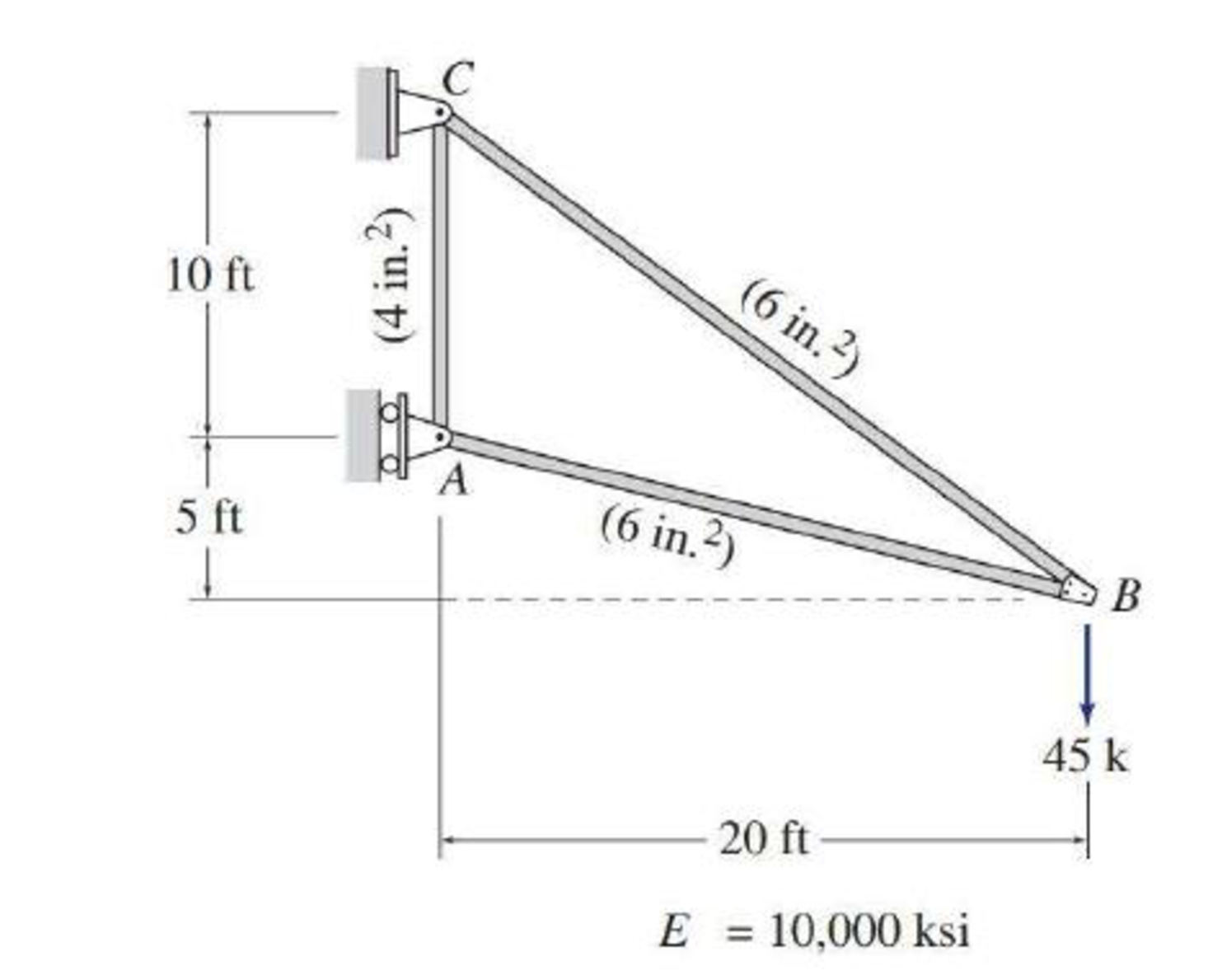# Use the virtual work method to determine the horizontal and vertical components of the deflection at joint B of the truss shown in Figs. P7.1–P7.5.

#### Solutions

Chapter
Section
Chapter 7, Problem 4P
Textbook Problem
84 views

## Use the virtual work method to determine the horizontal and vertical components of the deflection at joint B of the truss shown in Figs. P7.1–P7.5.To determine

Calculate the horizontal deflection and vertical deflection at joint B.

### Explanation of Solution

Given information:

The truss is given in the Figure.

The value of E is 10,000 ksi.

Procedure to find the deflection of truss by virtual work method is shown below.

• For Real system: If the deflection of truss is determined by the external loads, then apply method of joints or method of sections to find the real axial forces (F) in all the members of the truss.
• For virtual system: Remove all given real loads, apply a unit load at the joint where is deflection is required and also in the direction of desired deflection. Use method of joints or method of sections to find the virtual axial forces (Fv) in all the member of the truss.
• Finally use the desired deflection equation.

Apply the sign conventions for calculating reactions, forces and moments using the three equations of equilibrium as shown below.

• For summation of forces along x-direction is equal to zero (Fx=0), consider the forces acting towards right side as positive (+) and the forces acting towards left side as negative ().
• For summation of forces along y-direction is equal to zero (Fy=0), consider the upward force as positive (+) and the downward force as negative ().
• For summation of moment about a point is equal to zero (Matapoint=0), consider the clockwise moment as negative and the counter clockwise moment as positive.

Method of joints:

The negative value of force in any member indicates compression (C) and the positive value of force in any member indicates tension (T).

Condition for zero force members:

1. 1. If only two non-collinear members are connected to a joint that has no external loads or reactions applied to it, then the force in both the members is zero.
2. 2. If three members, two of which are collinear are connected to a joint that has no external loads or reactions applied to it, then the force in non-collinear member is zero.

Calculation:

Consider the real system.

Find the member axial force (F) for the real system using method of joints:

Let Cx and Cy be the horizontal and vertical reactions at the hinged support C.

Let Ax be the horizontal reaction at the roller support A.

Sketch the resultant diagram of the truss as shown in Figure 1.

Find the reactions at the supports using equilibrium equations:

Summation of moments about C is equal to 0.

MC=0Ax(10)45(20)=0Ax=90k

Summation of forces along y-direction is equal to 0.

+Fy=0Cy45=0Cy=45k

Summation of forces along x-direction is equal to 0.

+Fx=0AxCx=090Cx=0Cx=90k

Find the member forces using method of joints:

Apply equilibrium equation to the joint A:

Summation of forces along x-direction is equal to 0.

+Fx=090+FABcos14.04°=0FAB=92.77k

Summation of forces along y-direction is equal to 0.

+Fy=0FACFABsin14.04°=0FAC(92.77)sin14.04°=0FAC=22.5k

Apply equilibrium equation to the joint C:

Summation of forces along x-direction is equal to 0.

+Fx=090+FBCcos36.87°=0FBC=112.5k

Consider the virtual system:

For horizontal deflection apply l k at joint B in horizontal direction.

Find the member axial force (Fv1 ) due to virtual horizontal load using method of joints:

Sketch the resultant diagram of the virtual system (Fv1) as shown in Figure 2.

Find the reactions at the supports using equilibrium equations:

Summation of moments about C is equal to 0.

MC=0Ax(10)1(15)=0Ax=1.5k

Summation of forces along y-direction is equal to 0.

+Fy=0Cy=0

Summation of forces along x-direction is equal to 0.

+Fx=0Ax+Cx+1=01.5+Cx+1=0Cx=0.5k

Find the member forces of the truss using method of joints:

Apply equilibrium equation to the joint A:

Summation of forces along x-direction is equal to 0.

+Fx=01.5+FABcos14.04°=0FAB=1.546k

Summation of forces along y-direction is equal to 0.

+Fy=0FACFABsin14.04°=0FAC(1.546)sin14.04°=0FAC=0.375k

Apply equilibrium equation to the joint C:

Summation of forces along x-direction is equal to 0.

+Fx=00.5+FBCcos36.87°=0FBC=0.625k

Consider the virtual system:

For vertical deflection apply l k at joint B in vertical direction.

Find the member axial force (Fv2) due to virtual vertical load using method of joints:

Sketch the resultant diagram of the virtual system (Fv2) as shown in Figure 3.

Find the reactions at the supports using equilibrium equations:

Summation of moments about C is equal to 0.

MC=0Ax(10)1(20)=0Ax=2k

Summation of forces along y-direction is equal to 0

### Still sussing out bartleby?

Check out a sample textbook solution.

See a sample solution

#### The Solution to Your Study Problems

Bartleby provides explanations to thousands of textbook problems written by our experts, many with advanced degrees!

Get Started

Find more solutions based on key concepts
What is a matrix?

Engineering Fundamentals: An Introduction to Engineering (MindTap Course List)

When is a table in 1NF?

Database Systems: Design, Implementation, & Management

Software Update Issues You have heard that new software updates are available for your operating system, but wh...

Enhanced Discovering Computers 2017 (Shelly Cashman Series) (MindTap Course List)

Derive the expression for the largest angle ? for which the homogeneous block stays in equilibrium.

International Edition---engineering Mechanics: Statics, 4th Edition

What is the difference between a detail report, a summary report, and an exception report?

Systems Analysis and Design (Shelly Cashman Series) (MindTap Course List)

Why is it necessary to have blotters on both sides of a wheel?

Precision Machining Technology (MindTap Course List)

What types of protection are available to protect the ears during welding?

Welding: Principles and Applications (MindTap Course List)

If your motherboard supports ECC DDR3 memory, can you substitute non-ECC DDR3 memory?

A+ Guide to Hardware (Standalone Book) (MindTap Course List)